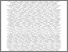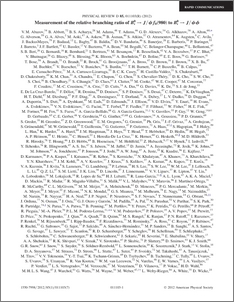# Measurement of the relative branching ratio of B^0_s to J/psi f_{0}(980) to B_{s}{0} to J/psi phi

collaboration, D0 and Bertram, Iain and Borissov, Guennadi and Fox, Harald and Ross, Anthony and Williams, Mark and Ratoff, Peter (2012) Measurement of the relative branching ratio of B^0_s to J/psi f_{0}(980) to B_{s}{0} to J/psi phi. Physical Review D, 85 (1). ISSN 1550-7998Preview
PDF
PhysRevD.85.011103.pdf - Published Version

## Abstract

We present a measurement of the relative branching fraction, R_{f_{0}/phi}, of B_{s}^{0} to J/\psi f_{0}(980), with f_{0}(980) to pi^+ pi^-, to the process B_{s}^{0} to J/psi phi, with phi to K^+K^-. The J/psi f_{0}(980) final state corresponds to a CP-odd eigenstate of B_{s}^{0} that could be of interest in future studies of CP violation. Using approximately 8fb^-1 of data recorded with the D0 detector at the Fermilab Tevatron Collider, we find R_{f_{0}/phi} = 0.275 +- 0.041(stat) +- 0.061(syst).

Item Type:
Journal Article
Journal or Publication Title:
Physical Review D